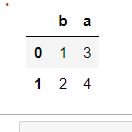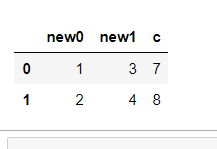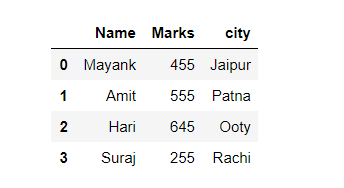# Rename column by index in Pandas

• Last Updated : 26 Dec, 2020

Prerequisites: Pandas

A column of a data frame can be changed using the position it is in known as its index. Just by the use of index a column can be renamed. This article discusses all such possible methods.

### Approach:

• Import required python library.
• Create data
• Provide index of the column to be renamed as argument to rename() function.

Pandas rename() method is used to rename any index, column or row.

Syntax: rename(mapper=None, index=None, columns=None, axis=None, copy=True, inplace=False, level=None)

Parameters:

• mapper, index and columns: Dictionary value, key refers to the old name and value refers to new name. Only one of these parameters can be used at once.
• axis: int or string value, 0/’row’ for Rows and 1/’columns’ for Columns
• copy: Copies underlying data if True.
• inplace: Makes changes in original Data Frame if True.
• level: Used to specify level in case data frame is having multiple level index.

Return Type: Data frame with new names

Given below are various implementation to achieve our required functionality:

Example 1: Switching both column names with each other using column index.

## Python3

 `import` `pandas as pd`` ` `# Sample DataFrame``df ``=` `pd.DataFrame({``'a'``: [``1``, ``2``], ``'b'``: [``3``, ``4``]})`` ` `# Changing columns name with index number``df.columns.values[``0``] ``=` `"b"``df.columns.values[``1``] ``=` `"a"`` ` `# Display``display(df)`

Output:Example 2: Using another method to rename the column with index.

## Python3

 `import` `pandas as pd`` ` `# Sample DataFrame``df ``=` `pd.DataFrame({``'a'``: [``1``, ``2``], ``'b'``: [``3``, ``4``]})`` ` `# Changing columns name with index number``su ``=` `df.rename(columns``=``{df.columns[``1``]: ``'new'``})`` ` `# Display``display(su)`

Output:Example 3: Renaming two/more columns in a single command using an index number.

## Python3

 `import` `pandas as pd`` ` `# Sample DataFrame``df ``=` `pd.DataFrame({``'a'``: [``1``, ``2``], ``'b'``: [``3``, ``4``], ``'c'``: [``7``, ``8``]})`` ` `# Changing columns name with index number``mapping ``=` `{df.columns[``0``]: ``'new0'``, df.columns[``1``]: ``'new1'``}``su ``=` `df.rename(columns``=``mapping)`` ` `# Display``display(su)`

Output:Example 4: Renaming column name with an index number of the CSV file.

File in use: Data1.csv

 `import` `pandas as pd`` ` `# reading a csv file``df1 ``=` `pd.read_csv(``"data1.csv"``)`` ` `# change 2nd column name with index number``df1.columns.values[``2``] ``=` `"city"`` ` `# Display DataFrame``display(df1)`